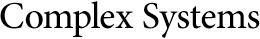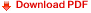## The Hydrodynamical Description for a Discrete Velocity Model of GasReneé Gatignol
Laboratoire de Mecanique Theorique,
U.A. 229 associée au C.N.R.S., Université Pierre et Marie Curie,
4 place Jussieu, 75252 Paris Cedex 05, France

#### Abstract

For a model of gas composed of identical particles with velocities restricted to a given finite set of vectors, the Boltzmann equation is replaced by a system of nonlinear coupled differential equations. The Chapman-Enskog method can be applied, and it gives the Navier-Stokes equations associated to the model. For the general model, we show that the dissipative terms in the Navier-Stokes equations do not depend on the mean number density nor on its gradient. For a gas near a homogeneous state, we give the transport coefficients explicitly.